# RD Sharma Solutions for Class 6 Maths Chapter 6: Fractions Exercise 6.5

Scoring good marks in Mathematics becomes easy with the help of RD Sharma Solutions Class 6 created by subject matter experts at BYJU’S. They prepare answers after conducting wide research on each concept to make them student friendly. This is the fifth exercise of Chapter 6 which provides a brief idea about equivalent fractions and methods of finding them. Solving exercise wise problems using the solutions PDF help students improve their speed. The students can download the RD Sharma Solutions for Class 6 Maths Chapter 6 Fractions Exercise 6.5 which are available here.

## RD Sharma Solutions for Class 6 Maths Chapter 6: Fractions Exercise 6.5 Download PDF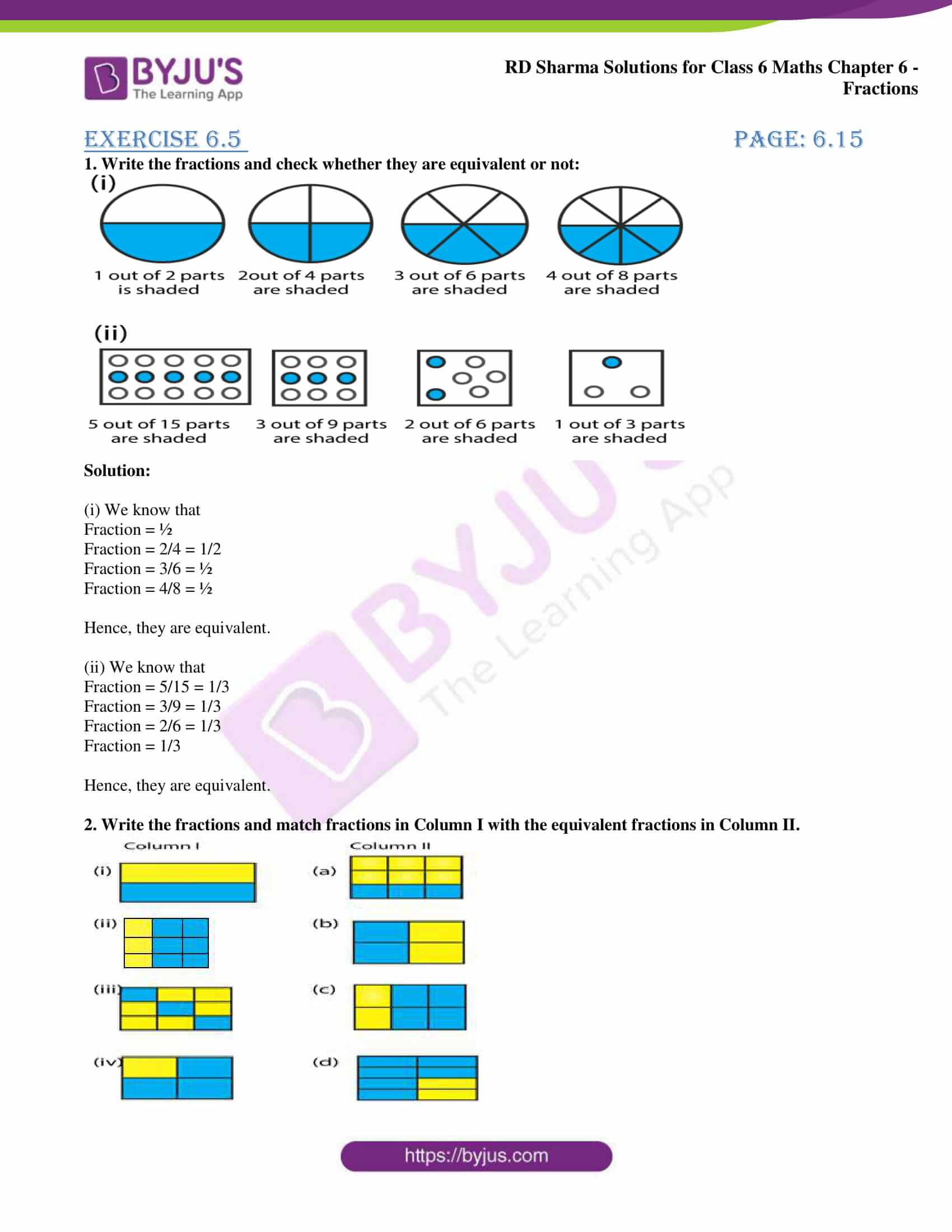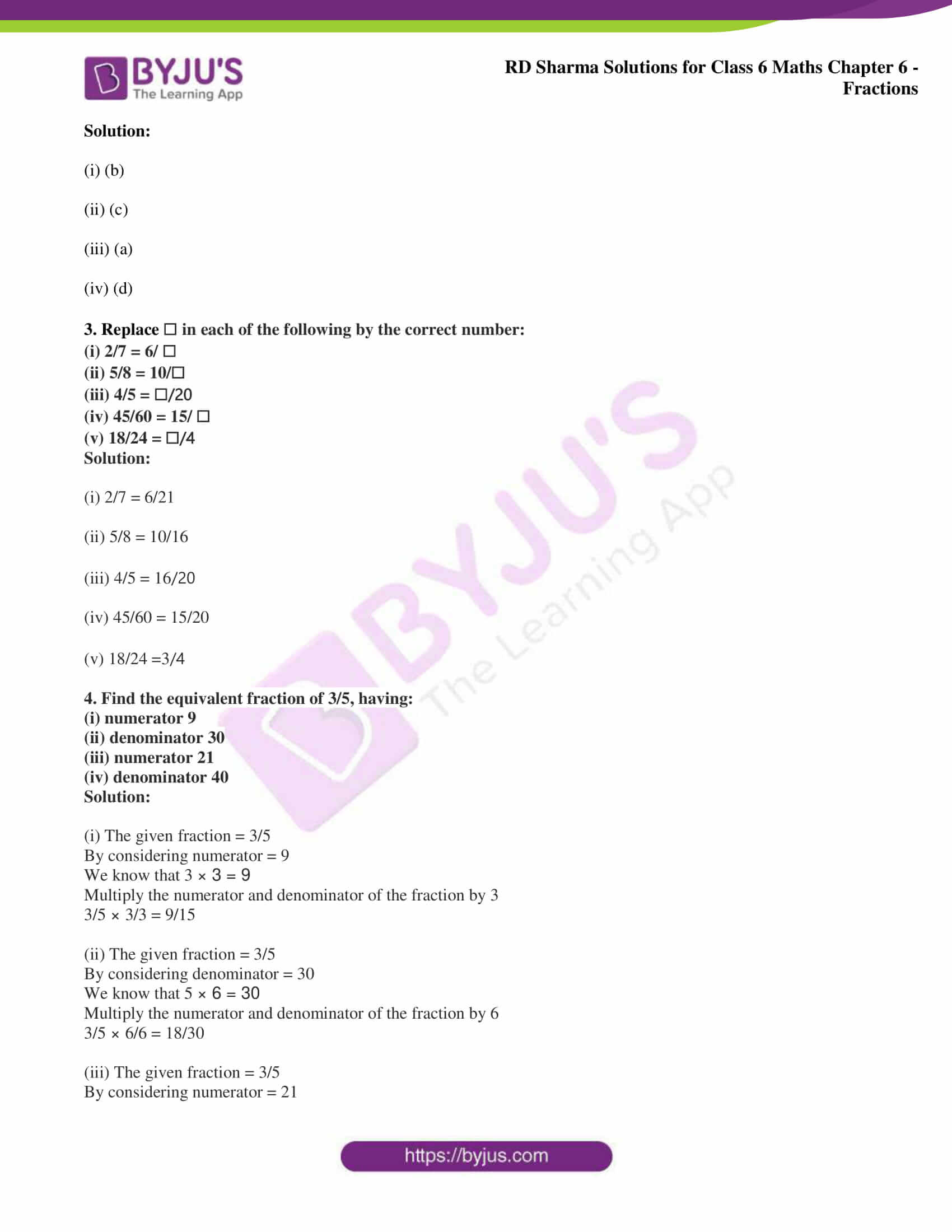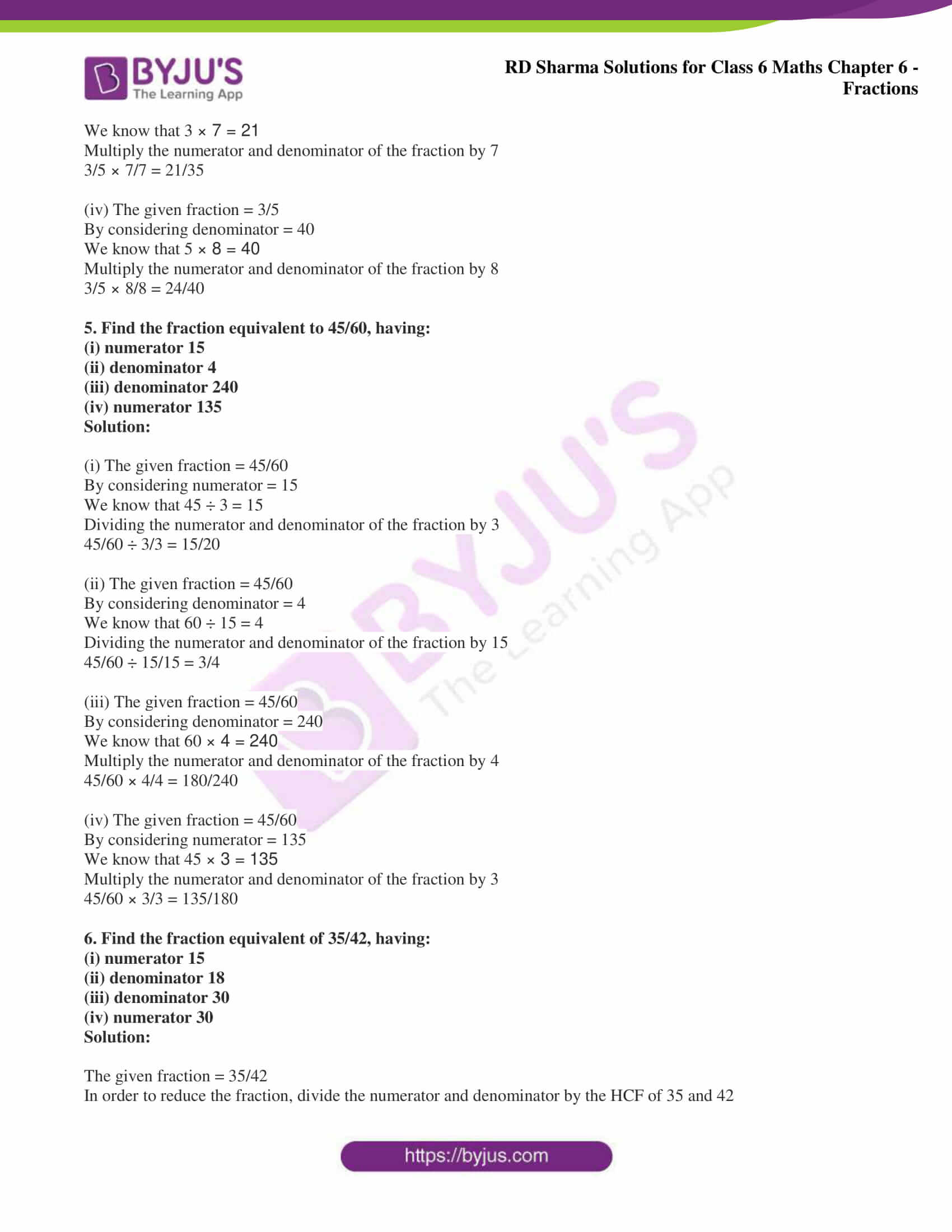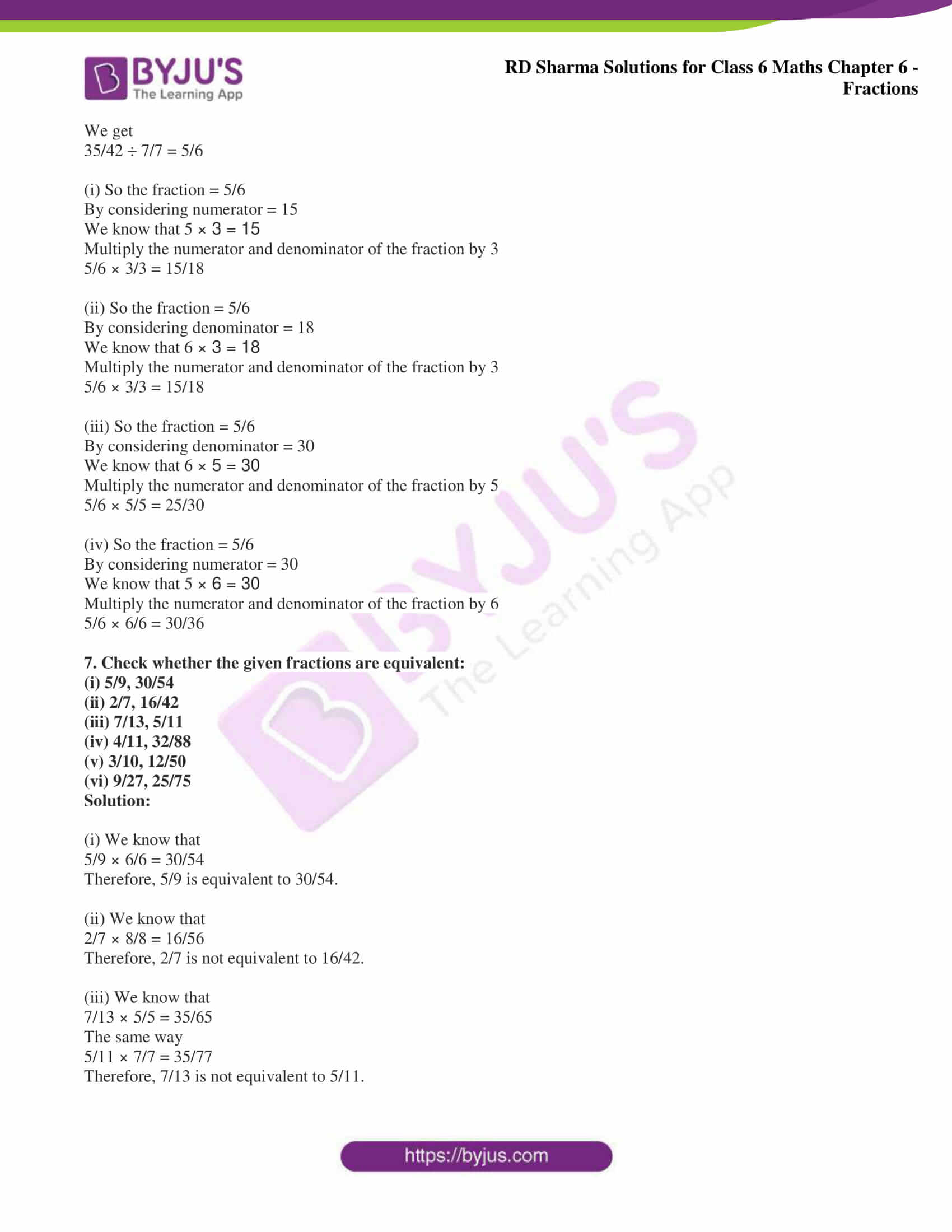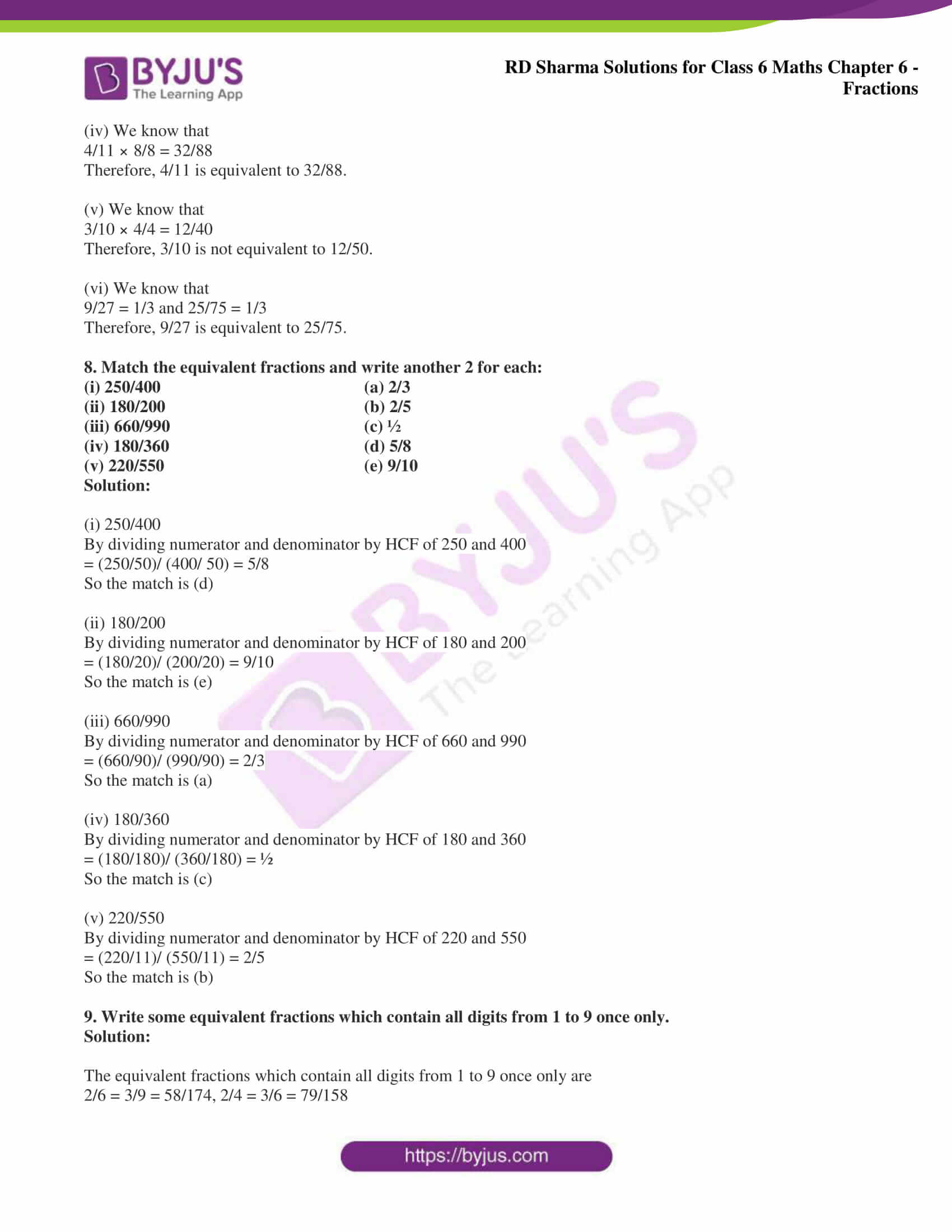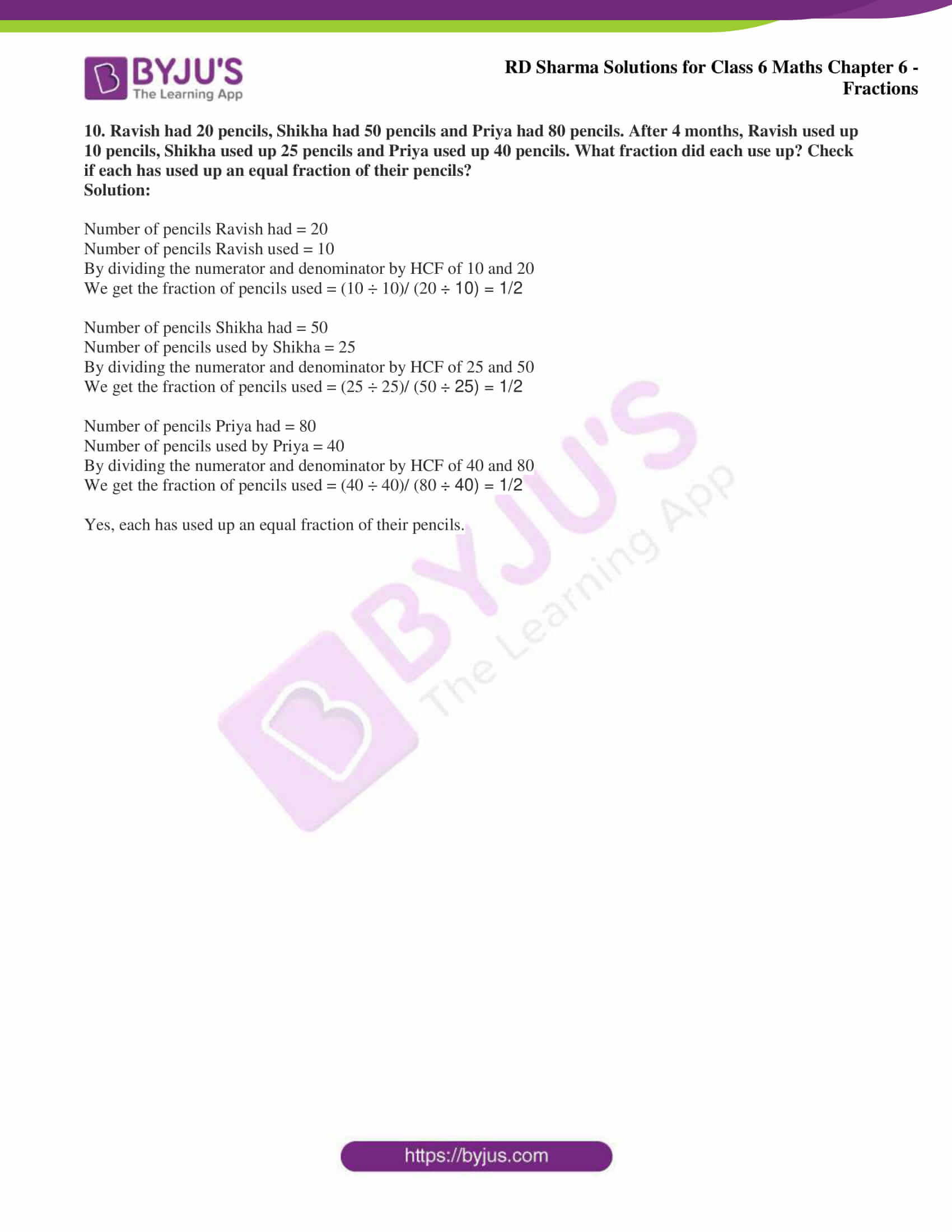### Access answers to Maths RD Sharma Solutions for Class 6 Chapter 6: Fractions Exercise 6.5

1. Write the fractions and check whether they are equivalent or not: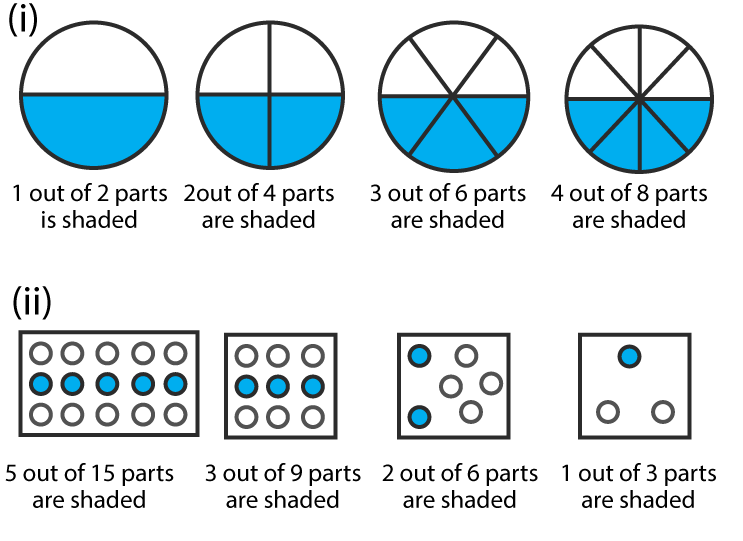Solution:

(i) We know that

Fraction = ½

Fraction = 2/4 = 1/2

Fraction = 3/6 = ½

Fraction = 4/8 = ½

Hence, they are equivalent.

(ii) We know that

Fraction = 5/15 = 1/3

Fraction = 3/9 = 1/3

Fraction = 2/6 = 1/3

Fraction = 1/3

Hence, they are equivalent.

2. Write the fractions and match fractions in Column I with the equivalent fractions in Column II.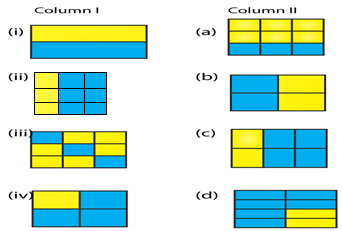Solution:

(i) (b)

(ii) (c)

(iii) (a)

(iv) (d)

3. Replace ☐ in each of the following by the correct number:

(i) 2/7 = 6/ ☐

(ii) 5/8 = 10/☐

(iii) 4/5 = ☐/20

(iv) 45/60 = 15/ ☐

(v) 18/24 = ☐/4

Solution:

(i) 2/7 = 6/21

(ii) 5/8 = 10/16

(iii) 4/5 = 16/20

(iv) 45/60 = 15/20

(v) 18/24 =3/4

4. Find the equivalent fraction of 3/5, having:

(i) numerator 9

(ii) denominator 30

(iii) numerator 21

(iv) denominator 40

Solution:

(i) The given fraction = 3/5

By considering numerator = 9

We know that 3 × 3 = 9

Multiply the numerator and denominator of the fraction by 3

3/5 × 3/3 = 9/15

(ii) The given fraction = 3/5

By considering denominator = 30

We know that 5 × 6 = 30

Multiply the numerator and denominator of the fraction by 6

3/5 × 6/6 = 18/30

(iii) The given fraction = 3/5

By considering numerator = 21

We know that 3 × 7 = 21

Multiply the numerator and denominator of the fraction by 7

3/5 × 7/7 = 21/35

(iv) The given fraction = 3/5

By considering denominator = 40

We know that 5 × 8 = 40

Multiply the numerator and denominator of the fraction by 8

3/5 × 8/8 = 24/40

5. Find the fraction equivalent to 45/60, having:

(i) numerator 15

(ii) denominator 4

(iii) denominator 240

(iv) numerator 135

Solution:

(i) The given fraction = 45/60

By considering numerator = 15

We know that 45 ÷ 3 = 15

Dividing the numerator and denominator of the fraction by 3

45/60 ÷ 3/3 = 15/20

(ii) The given fraction = 45/60

By considering denominator = 4

We know that 60 ÷ 15 = 4

Dividing the numerator and denominator of the fraction by 15

45/60 ÷ 15/15 = 3/4

(iii) The given fraction = 45/60

By considering denominator = 240

We know that 60 × 4 = 240

Multiply the numerator and denominator of the fraction by 4

45/60 × 4/4 = 180/240

(iv) The given fraction = 45/60

By considering numerator = 135

We know that 45 × 3 = 135

Multiply the numerator and denominator of the fraction by 3

45/60 × 3/3 = 135/180

6. Find the fraction equivalent of 35/42, having:

(i) numerator 15

(ii) denominator 18

(iii) denominator 30

(iv) numerator 30

Solution:

The given fraction = 35/42

In order to reduce the fraction, divide the numerator and denominator by the HCF of 35 and 42

We get

35/42 ÷ 7/7 = 5/6

(i) So the fraction = 5/6

By considering numerator = 15

We know that 5 × 3 = 15

Multiply the numerator and denominator of the fraction by 3

5/6 × 3/3 = 15/18

(ii) So the fraction = 5/6

By considering denominator = 18

We know that 6 × 3 = 18

Multiply the numerator and denominator of the fraction by 3

5/6 × 3/3 = 15/18

(iii) So the fraction = 5/6

By considering denominator = 30

We know that 6 × 5 = 30

Multiply the numerator and denominator of the fraction by 5

5/6 × 5/5 = 25/30

(iv) So the fraction = 5/6

By considering numerator = 30

We know that 5 × 6 = 30

Multiply the numerator and denominator of the fraction by 6

5/6 × 6/6 = 30/36

7. Check whether the given fractions are equivalent:

(i) 5/9, 30/54

(ii) 2/7, 16/42

(iii) 7/13, 5/11

(iv) 4/11, 32/88

(v) 3/10, 12/50

(vi) 9/27, 25/75

Solution:

(i) We know that

5/9 × 6/6 = 30/54

Therefore, 5/9 is equivalent to 30/54.

(ii) We know that

2/7 × 8/8 = 16/56

Therefore, 2/7 is not equivalent to 16/42.

(iii) We know that

7/13 × 5/5 = 35/65

The same way

5/11 × 7/7 = 35/77

Therefore, 7/13 is not equivalent to 5/11.

(iv) We know that

4/11 × 8/8 = 32/88

Therefore, 4/11 is equivalent to 32/88.

(v) We know that

3/10 × 4/4 = 12/40

Therefore, 3/10 is not equivalent to 12/50.

(vi) We know that

9/27 = 1/3 and 25/75 = 1/3

Therefore, 9/27 is equivalent to 25/75.

8. Match the equivalent fractions and write another 2 for each:

(i) 250/400 (a) 2/3

(ii) 180/200 (b) 2/5

(iii) 660/990 (c) ½

(iv) 180/360 (d) 5/8

(v) 220/550 (e) 9/10

Solution:

(i) 250/400

By dividing numerator and denominator by HCF of 250 and 400

= (250/50)/ (400/ 50) = 5/8

So the match is (d)

(ii) 180/200

By dividing numerator and denominator by HCF of 180 and 200

= (180/20)/ (200/20) = 9/10

So the match is (e)

(iii) 660/990

By dividing numerator and denominator by HCF of 660 and 990

= (660/90)/ (990/90) = 2/3

So the match is (a)

(iv) 180/360

By dividing numerator and denominator by HCF of 180 and 360

= (180/180)/ (360/180) = ½

So the match is (c)

(v) 220/550

By dividing numerator and denominator by HCF of 220 and 550

= (220/11)/ (550/11) = 2/5

So the match is (b)

9. Write some equivalent fractions which contain all digits from 1 to 9 once only.

Solution:

The equivalent fractions which contain all digits from 1 to 9 once only are

2/6 = 3/9 = 58/174, 2/4 = 3/6 = 79/158

10. Ravish had 20 pencils, Shikha had 50 pencils and Priya had 80 pencils. After 4 months, Ravish used up 10 pencils, Shikha used up 25 pencils and Priya used up 40 pencils. What fraction did each use up? Check if each has used up an equal fraction of their pencils?

Solution:

Number of pencils Ravish had = 20

Number of pencils Ravish used = 10

By dividing the numerator and denominator by HCF of 10 and 20

We get the fraction of pencils used = (10 ÷ 10)/ (20 ÷ 10) = 1/2

Number of pencils Shikha had = 50

Number of pencils used by Shikha = 25

By dividing the numerator and denominator by HCF of 25 and 50

We get the fraction of pencils used = (25 ÷ 25)/ (50 ÷ 25) = 1/2

Number of pencils Priya had = 80

Number of pencils used by Priya = 40

By dividing the numerator and denominator by HCF of 40 and 80

We get the fraction of pencils used = (40 ÷ 40)/ (80 ÷ 40) = 1/2

Yes, each has used up an equal fraction of their pencils.